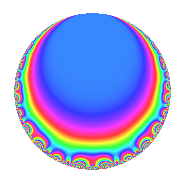# Properties

 Label 672.2.q.hLevel 672 Weight 2 Character orbit 672.q Analytic conductor 5.366 Analytic rank 0 Dimension 2 CM No Inner twists 2

# Related objects

## Newspace parameters

 Level: $$N$$ = $$672 = 2^{5} \cdot 3 \cdot 7$$ Weight: $$k$$ = $$2$$ Character orbit: $$[\chi]$$ = 672.q (of order $$3$$ and degree $$2$$)

## Newform invariants

 Self dual: No Analytic conductor: $$5.36594701583$$ Analytic rank: $$0$$ Dimension: $$2$$ Coefficient field: $$\Q(\sqrt{-3})$$ Coefficient ring: $$\Z[a_1, a_2, a_3]$$ Coefficient ring index: $$1$$ Sato-Tate group: $\mathrm{SU}(2)[C_{3}]$

## $q$-expansion

Coefficients of the $$q$$-expansion are expressed in terms of a primitive root of unity $$\zeta_{6}$$. We also show the integral $$q$$-expansion of the trace form.

 $$f(q)$$ $$=$$ $$q + ( 1 - \zeta_{6} ) q^{3} + ( -2 - \zeta_{6} ) q^{7} -\zeta_{6} q^{9} +O(q^{10})$$ $$q + ( 1 - \zeta_{6} ) q^{3} + ( -2 - \zeta_{6} ) q^{7} -\zeta_{6} q^{9} + ( 2 - 2 \zeta_{6} ) q^{11} + q^{13} + ( 2 - 2 \zeta_{6} ) q^{17} -5 \zeta_{6} q^{19} + ( -3 + 2 \zeta_{6} ) q^{21} -6 \zeta_{6} q^{23} + ( 5 - 5 \zeta_{6} ) q^{25} - q^{27} -8 q^{29} + ( 3 - 3 \zeta_{6} ) q^{31} -2 \zeta_{6} q^{33} + 9 \zeta_{6} q^{37} + ( 1 - \zeta_{6} ) q^{39} + 2 q^{41} + q^{43} -8 \zeta_{6} q^{47} + ( 3 + 5 \zeta_{6} ) q^{49} -2 \zeta_{6} q^{51} + ( -6 + 6 \zeta_{6} ) q^{53} -5 q^{57} + ( -6 + 6 \zeta_{6} ) q^{59} + 2 \zeta_{6} q^{61} + ( -1 + 3 \zeta_{6} ) q^{63} + ( 5 - 5 \zeta_{6} ) q^{67} -6 q^{69} + 4 q^{71} + ( 11 - 11 \zeta_{6} ) q^{73} -5 \zeta_{6} q^{75} + ( -6 + 4 \zeta_{6} ) q^{77} + 5 \zeta_{6} q^{79} + ( -1 + \zeta_{6} ) q^{81} + ( -8 + 8 \zeta_{6} ) q^{87} -12 \zeta_{6} q^{89} + ( -2 - \zeta_{6} ) q^{91} -3 \zeta_{6} q^{93} + 18 q^{97} -2 q^{99} +O(q^{100})$$ $$\operatorname{Tr}(f)(q)$$ $$=$$ $$2q + q^{3} - 5q^{7} - q^{9} + O(q^{10})$$ $$2q + q^{3} - 5q^{7} - q^{9} + 2q^{11} + 2q^{13} + 2q^{17} - 5q^{19} - 4q^{21} - 6q^{23} + 5q^{25} - 2q^{27} - 16q^{29} + 3q^{31} - 2q^{33} + 9q^{37} + q^{39} + 4q^{41} + 2q^{43} - 8q^{47} + 11q^{49} - 2q^{51} - 6q^{53} - 10q^{57} - 6q^{59} + 2q^{61} + q^{63} + 5q^{67} - 12q^{69} + 8q^{71} + 11q^{73} - 5q^{75} - 8q^{77} + 5q^{79} - q^{81} - 8q^{87} - 12q^{89} - 5q^{91} - 3q^{93} + 36q^{97} - 4q^{99} + O(q^{100})$$

## Character Values

We give the values of $$\chi$$ on generators for $$\left(\mathbb{Z}/672\mathbb{Z}\right)^\times$$.

 $$n$$ $$127$$ $$421$$ $$449$$ $$577$$ $$\chi(n)$$ $$1$$ $$1$$ $$1$$ $$-\zeta_{6}$$

## Embeddings

For each embedding $$\iota_m$$ of the coefficient field, the values $$\iota_m(a_n)$$ are shown below.

For more information on an embedded modular form you can click on its label.

Label $$\iota_m(\nu)$$ $$a_{2}$$ $$a_{3}$$ $$a_{4}$$ $$a_{5}$$ $$a_{6}$$ $$a_{7}$$ $$a_{8}$$ $$a_{9}$$ $$a_{10}$$
193.1
 0.5 + 0.866025i 0.5 − 0.866025i
0 0.500000 0.866025i 0 0 0 −2.50000 0.866025i 0 −0.500000 0.866025i 0
289.1 0 0.500000 + 0.866025i 0 0 0 −2.50000 + 0.866025i 0 −0.500000 + 0.866025i 0
 $$n$$: e.g. 2-40 or 990-1000 Significant digits: Format: Complex embeddings Normalized embeddings Satake parameters Satake angles

## Inner twists

Char. orbit Parity Mult. Self Twist Proved
1.a Even 1 trivial yes
7.c Even 1 yes

## Hecke kernels

This newform can be constructed as the intersection of the kernels of the following linear operators acting on $$S_{2}^{\mathrm{new}}(672, [\chi])$$:

 $$T_{5}$$ $$T_{11}^{2} - 2 T_{11} + 4$$ $$T_{13} - 1$$